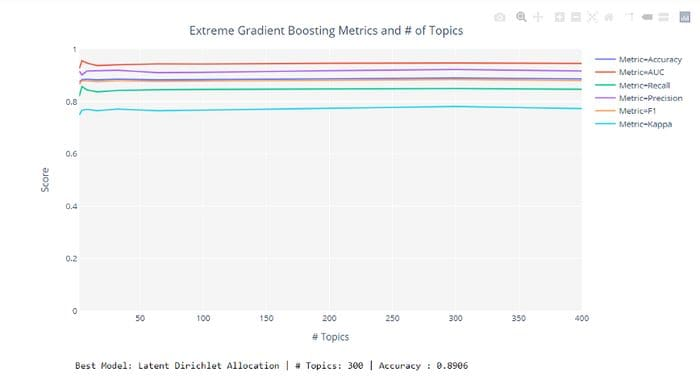### How to tune "n parameter" in unsupervised experiments

In unsupervised machine learning the "n parameter" i.e. the number of clusters for clustering experiments, the fraction of the outliers in anomaly detection, and the number of topics in topic modeling, is of fundamental importance.

When the eventual objective of the experiment is to predict an outcome (classification or regression) using the results from the unsupervised experiments, then the tune_model() function in the pycaret.clustering module the pycaret.anomaly module, and the pycaret.nlp module comes in very handy.

To understand this, let's see an example using the "Kiva" dataset.This is a micro-banking loan dataset where each row represents a borrower with their relevant information. Column 'en' captures the loan application text of each borrower, and the column 'status' represents whether the borrower defaulted or not (default = 1 or no default = 0).

You can use tune_model function in pycaret.nlp to optimize num_topics parameter based on the target variable of supervised experiment (i.e. predicting the optimum number of topics required to improve the prediction of the final target variable). You can define the model for training using estimator parameter ('xgboost' in this case). This function returns a trained topic model and a visual showing supervised metrics at each iteration.

`tuned_lda = tune_model(model='lda', supervised_target='status', estimator='xgboost')`#### More Articles of Aditi Kothiyal:

Name Views Likes
Python PyCaret How to optimize the probability threshold % in binary classification 2069 0
Python K-means Predicting Iris Flower Species 1322 2
Python PyCaret How to ignore certain columns for model building 2624 0
Python PyCaret Experiment Logging 680 0
Python PyWin32 Open a File in Excel 941 0
Python Guppy GSL Introduction 219 2
Python Usage of Guppy With Example 1101 2
Python Naive Bayes Tutorial 552 2
Python Guppy Recent Memory Usage of a Program 892 2
Python K-Means Clustering Applications 332 2
Python Random Forest Algorithm Decision Trees 439 0
Python K-means Clustering PREDICTING IRIS FLOWER SPECIES 457 1
Python Random Forest Algorithm Bootstrap 476 0
Python PyCaret Util Functions 441 0
Python K-means Music Genre Classification 1763 1
Python PyWin Attach an Excel file to Outlook 1541 0
Python Guppy GSL Document and Test Example 248 2
Python Random Forest Algorithm Bagging 386 0
Python PyWin32 Getting Started PyWin32 602 0
Python Naive Bayes in Machine Learning 374 2
Python PyCaret How to improve results from hyperparameter tuning by increasing "n_iter" 1723 0
Python PyCaret Getting Started with PyCaret 2.0 356 1
Python PyCaret Tune Model 1325 1
Python PyCaret Create your own AutoML software 321 0
Python PyCaret Intoduction to PyCaret 296 1
Python PyCaret Compare Models 2696 1
Python PyWin Copying Data into Excel 1153 0
Python Guppy Error: expected function body after function declarator 413 2
Python Coding Random forest classifier using xgBoost 247 0
Python PyCaret How to tune "n parameter" in unsupervised experiments 659 0
Python PyCaret How to programmatically define data types in the setup function 1403 0
Python PyCaret Ensemble Model 805 1
Python Random forest algorithm Introduction 227 0
Python k-means Clustering Example 337 1
Python PyCaret Plot Model 1243 1
Python Hamming Distance 715 0
Python Understanding Random forest algorithm 311 0
Python PyCaret Sort a Dictionary by Keys 244 0
Python Coding Random forest classifier using sklearn 340 0
Python Guppy Introduction 368 2
Python How to use Guppy/Heapy for tracking down Memory Usage 1069 2
Python AdaBoost Summary and Conclusion 232 1
Python PyCaret Create Model 365 1
Python k -means Clusturing Introduction 325 2
Python k-means Clustering With Example 348 2